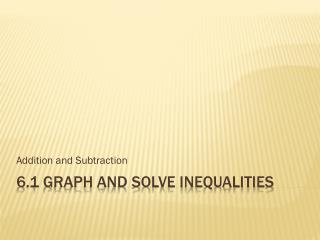# 6.1 Graph and Solve Inequalities - PowerPoint PPT PresentationDownload Presentation6.1 Graph and Solve Inequalities

Download Presentation## 6.1 Graph and Solve Inequalities

- - - - - - - - - - - - - - - - - - - - - - - - - - - E N D - - - - - - - - - - - - - - - - - - - - - - - - - - -
##### Presentation Transcript

1. Addition and Subtraction 6.1 Graph and Solve Inequalities

2. Next

3. Next

4. Next

5. Solve the inequality. Hint Solution

6. Solve the inequality. Solution Add 4 to both sides of the inequality.

7. Next

8. Solve the inequality. Hint Solution

9. Solve the inequality. Subtract 8 from both sides of the inequality. Solution

10. Next

11. Solve the inequality. Hint Solution

12. Solve the inequality. Subtract 10.2 from both sides of the inequality. Solution

13. Next

14. Solve the inequality. Hint Solution

15. Solve the inequality. Add to both sides of the inequality. Solution

16. Next

17. Solve the inequality. Hint Solution

18. Solve the inequality. Add 1.8 to both sides of the inequality. Solution

19. The End